# 教你实现微信8.0『炸裂』的🎉表情特效

20358

### 写在开头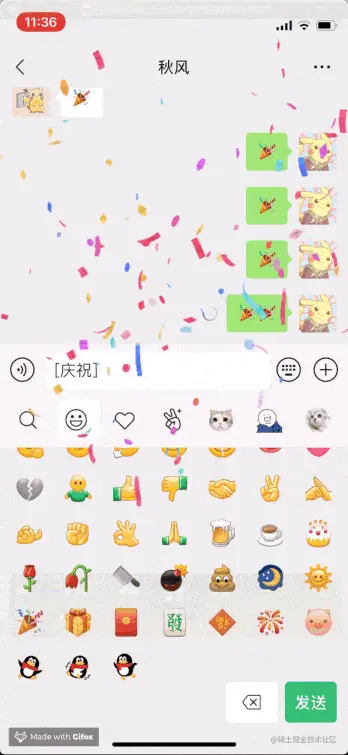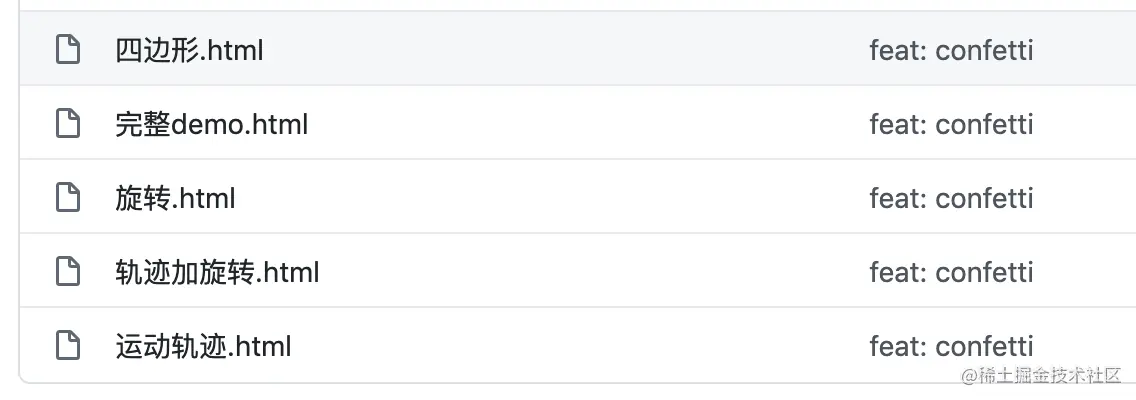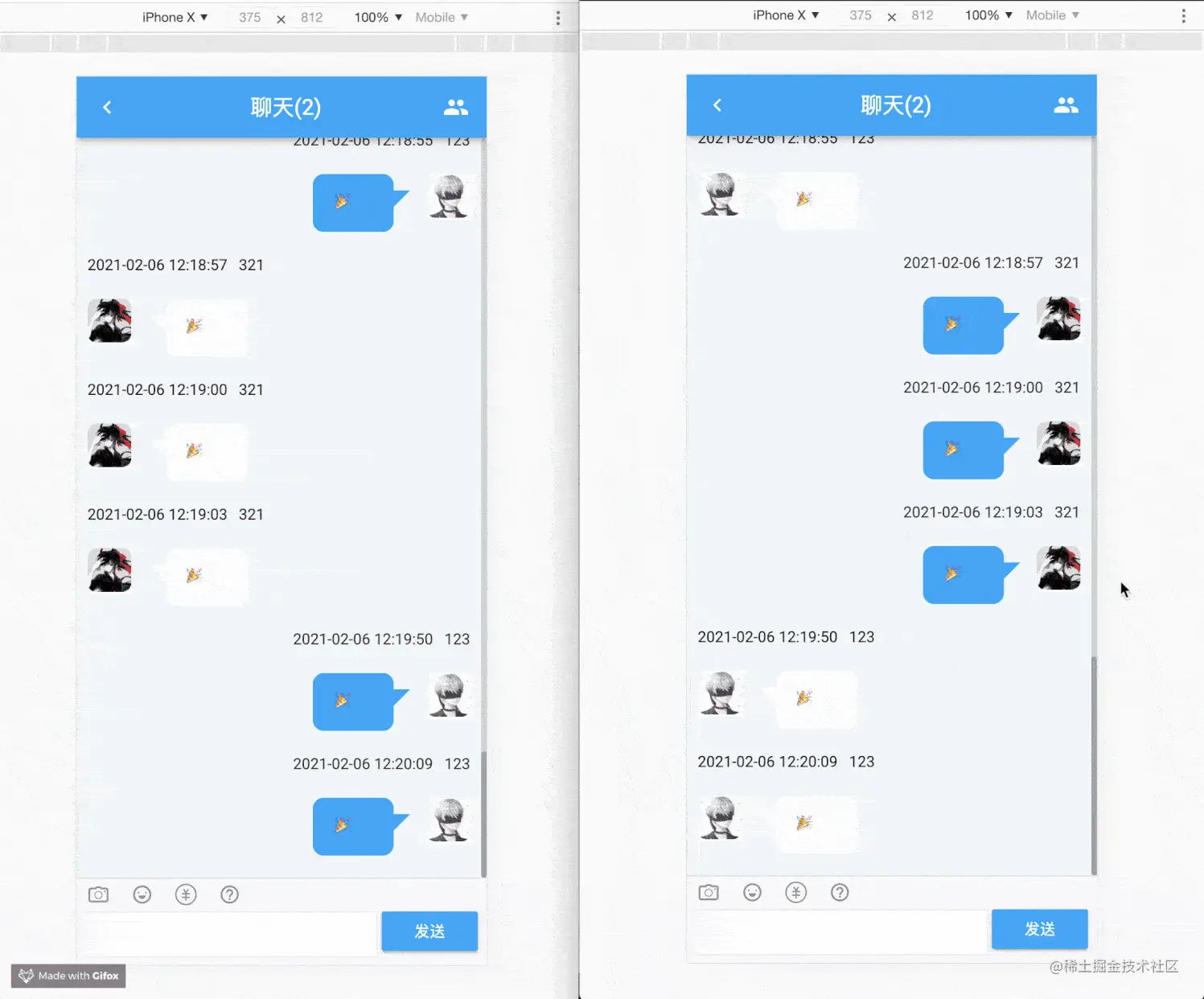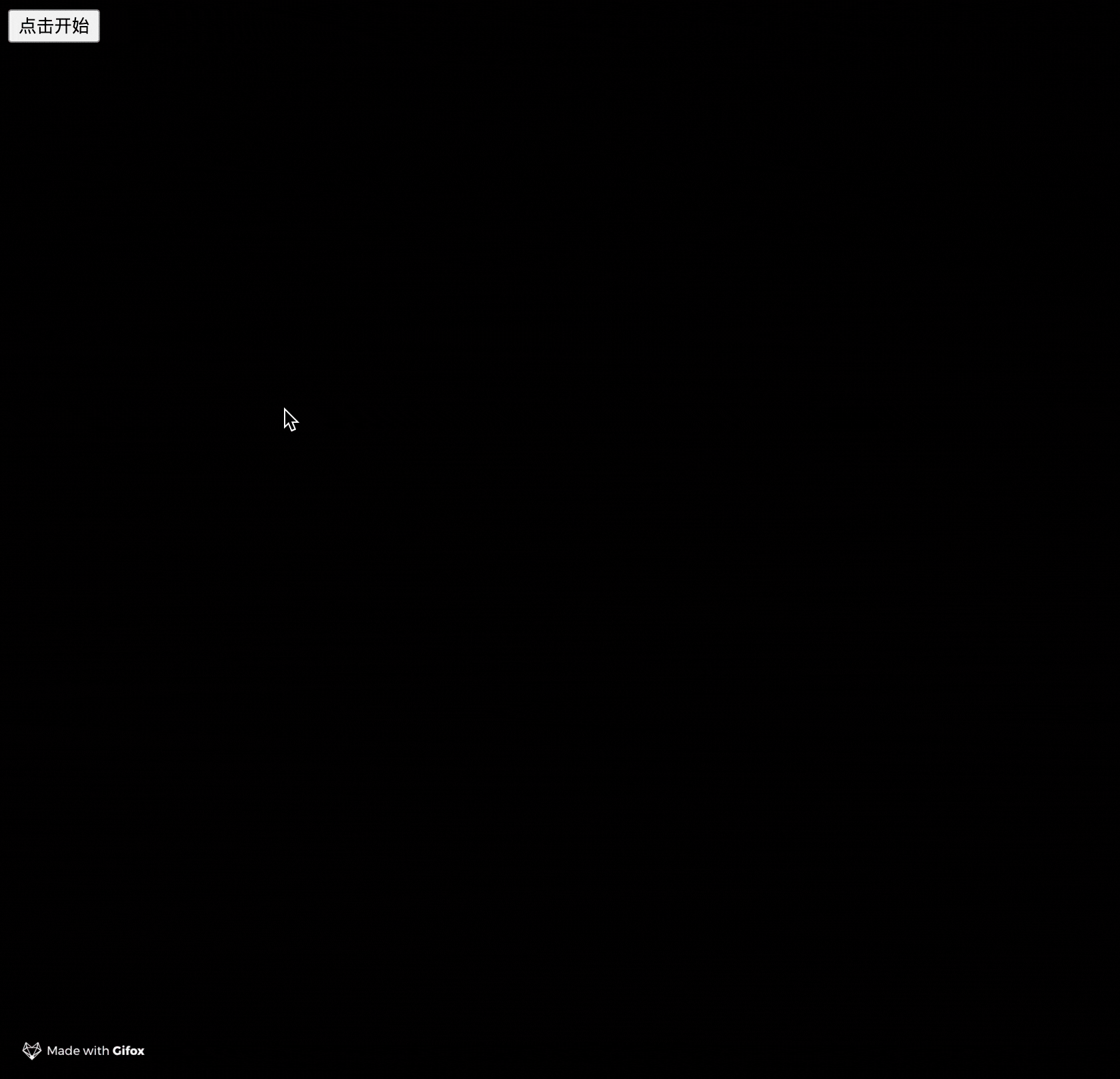### 前期研究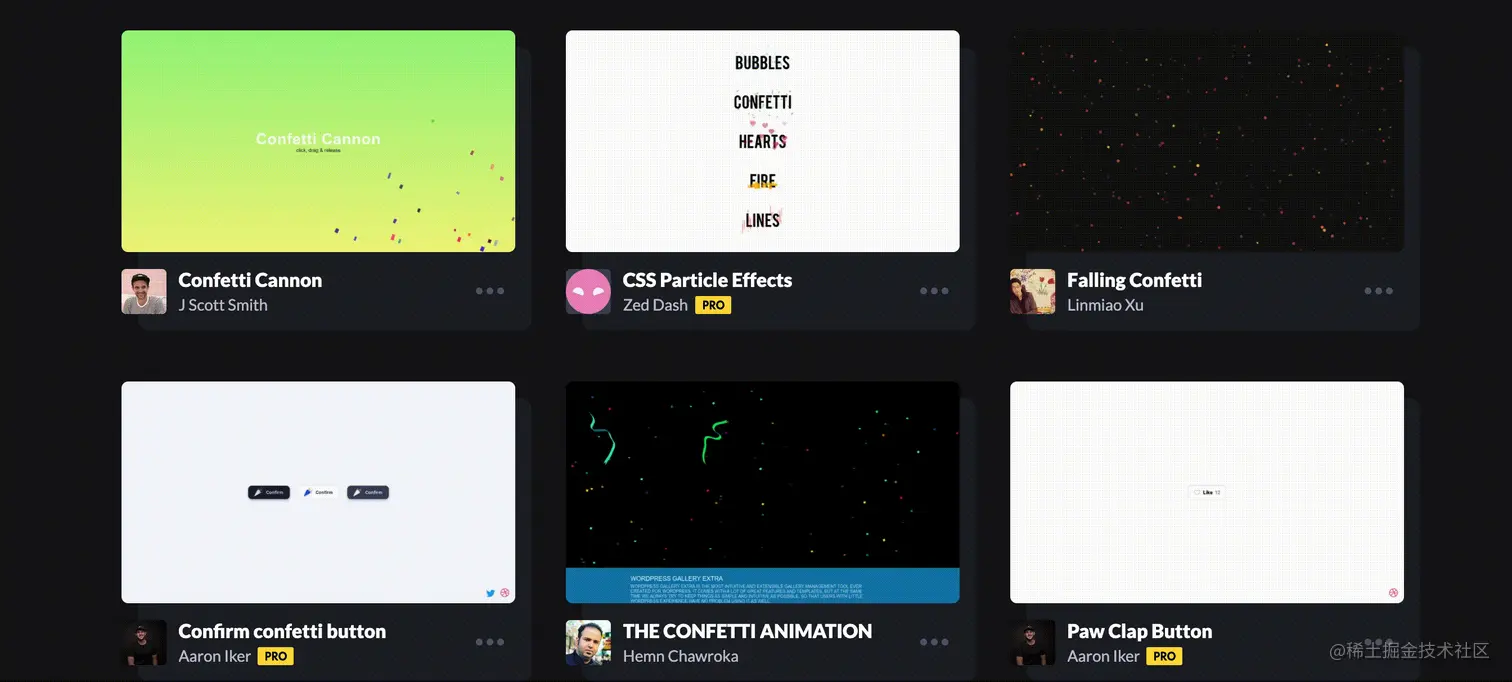### 核心实现

#### 切片场景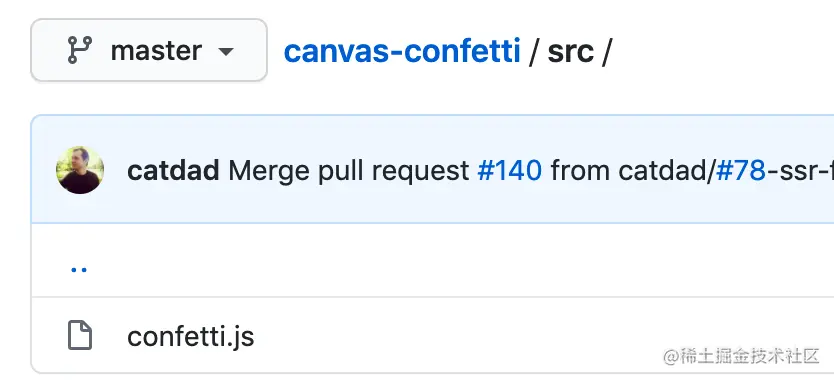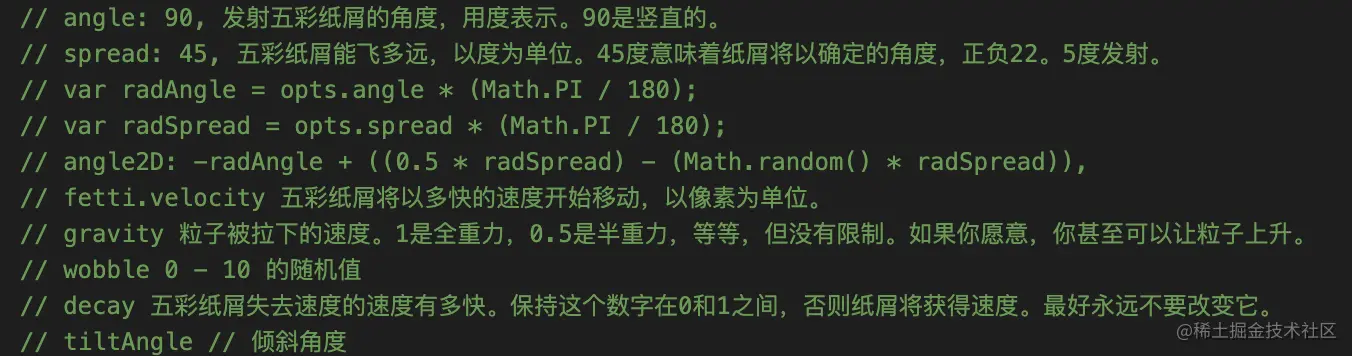``````fetti.x += Math.cos(fetti.angle2D) * fetti.velocity;
fetti.y += Math.sin(fetti.angle2D) * fetti.velocity + fetti.gravity;
``````

#### 平行四边形的实现

beginPath

moveTo

lineTo

closePath

fill

fillStyle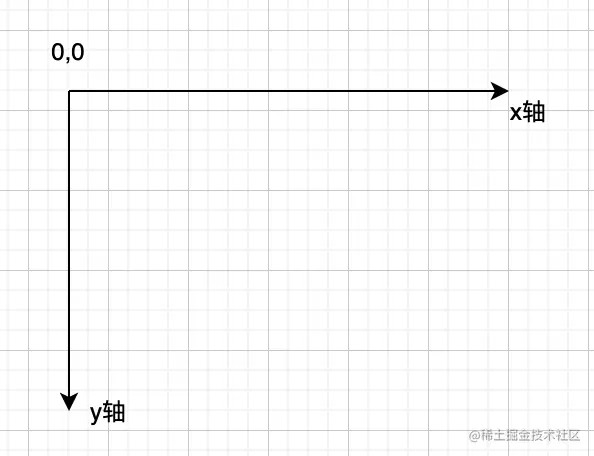``````...(省略了一些前置初始化代码)
var context = canvas.getContext('2d');
// 清除画布
context.clearRect(0, 0, canvas.width, canvas.height);
// 设置颜色并开始绘制
context.fillStyle = 'rgba(2, 255, 255, 1)';
context.beginPath();
// 设置几个点
var point1 = { x: 0, y: 0 }
var point2 = { x: 0, y: 20 }
var point3 = { x: 20, y: 20 }
var point4 = { x: 20, y: 0 }
// 画4个点
context.moveTo(Math.floor(point1.x), Math.floor(point1.y));
context.lineTo(Math.floor(point2.x), Math.floor(point2.y));
context.lineTo(Math.floor(point3.x), Math.floor(point3.y));
context.lineTo(Math.floor(point4.x), Math.floor(point4.y));
// 完成路线，并填充
context.closePath();
context.fill();
``````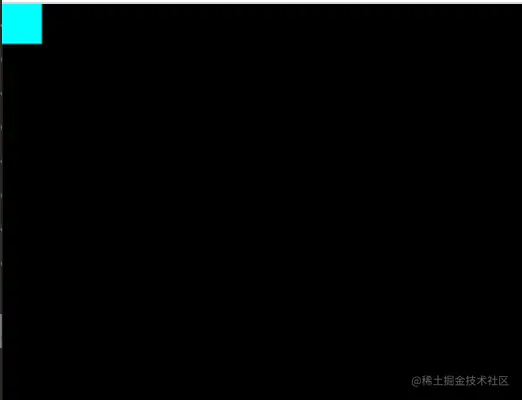#### 运动轨迹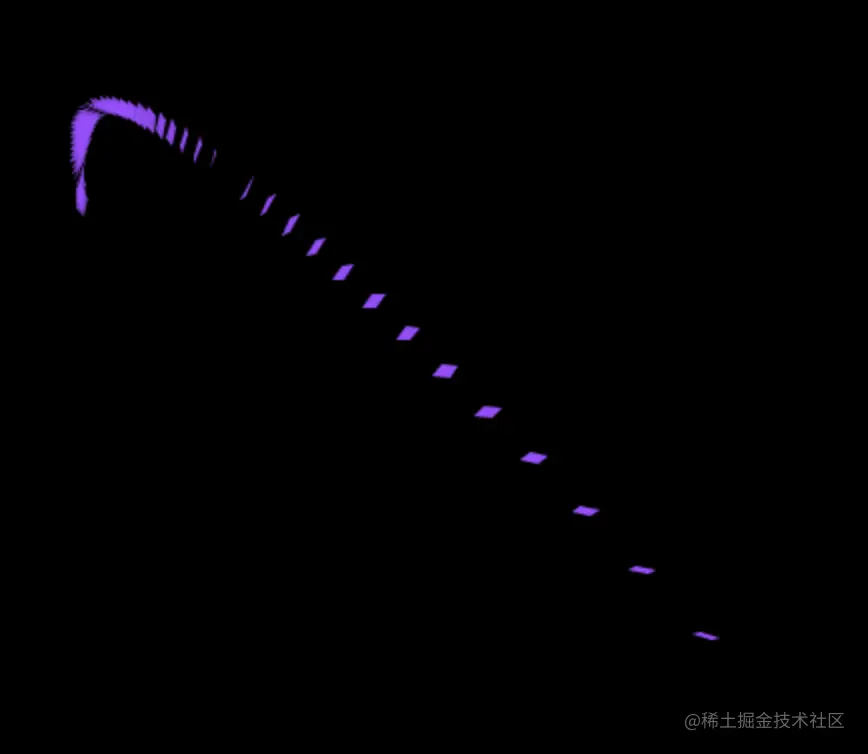``````// fetti.angle2D为一个角度（这个角度确定了运动轨迹 3 / 2 * Math.PI - 2 * Math.PI之间的一个值，由于要让轨迹往左上角移动，就是都要往负方向运动，因此选了以上范围），
// fetti.velocity 为一个初始为50长度的值。
// fetti.gravity = 3
fetti.x += Math.cos(fetti.angle2D) * fetti.velocity; // fetti.x 第一个点的x坐标
fetti.y += Math.sin(fetti.angle2D) * fetti.velocity + fetti.gravity; // fetti.y 第一个点的y坐标
fetti.velocity *= 0.8；
``````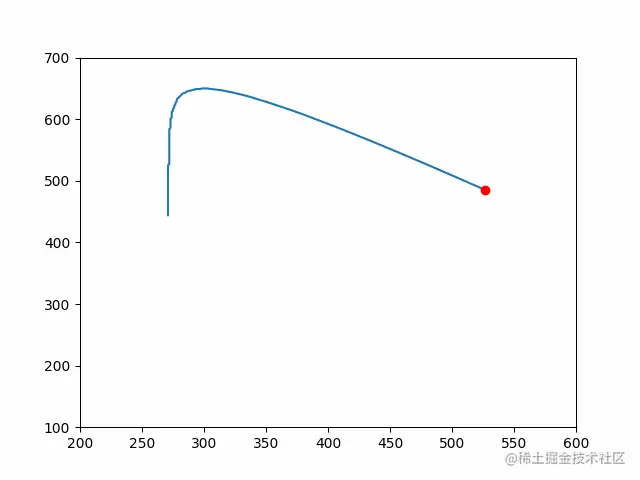``````const fetti = {
"x": 445,
"y": 541,
"angle2D": 3 / 2 * Math.PI + 1 / 6 * Math.PI,
"color": {r: 20, g: 30, b: 50},
"tick": 0,
"totalTicks": 200,
"decay": 0.9,
"gravity": 3,
"velocity": 50
}
var animationFrame = null;
const update = () => {
context.clearRect(0, 0, canvas.width, canvas.height);
context.fillStyle = 'rgba(2, 255, 255, 1)';
context.beginPath();
fetti.x += Math.cos(fetti.angle2D) * fetti.velocity; // 第一个点
fetti.y += Math.sin(fetti.angle2D) * fetti.velocity + fetti.gravity; // 第一个点

var x1 = fetti.x;
var y1 = fetti.y;

var x2 = fetti.x;// 第二个点
var y2 = fetti.y + 10; // 第二个点

var x3 = x1 + 10;
var y3 = y1 + 10;

var x4 = fetti.x + 10;
var y4 = fetti.y;

fetti.velocity *= fetti.decay;

context.moveTo(Math.floor(x1), Math.floor(y1));
context.lineTo(Math.floor(x2), Math.floor(y2));
context.lineTo(Math.floor(x3), Math.floor(y3));
context.lineTo(Math.floor(x4), Math.floor(y4));

context.closePath();
context.fill();
animationFrame = raf.frame(update);
}
``````#### 反转特效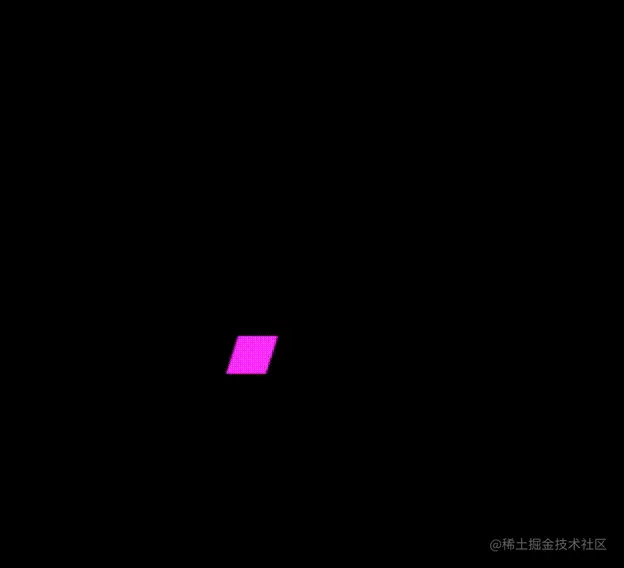• 知道一个点的位置

• 知道一个角度

• 知道一边边长

``````const update = () => {
context.clearRect(0, 0, canvas.width, canvas.height);
context.fillStyle = 'rgba(2, 255, 255, 1)';
context.beginPath();

fetti.velocity *= fetti.decay;
fetti.tiltAngle += 0.1 // 不断给这个四边形变化角度

var length = 10;

var x1 = fetti.x;
var y1 = fetti.y;

var x2 = fetti.x + (length * Math.sin(fetti.tiltAngle));// 第二个点
var y2 = fetti.y + (length * Math.cos(fetti.tiltAngle)); // 第二个点

var x3 = x2 + 10;
var y3 = y2;

var x4 = fetti.x + length;
var y4 = fetti.y;

context.moveTo(Math.floor(x1), Math.floor(y1));
context.lineTo(Math.floor(x2), Math.floor(y2));
context.lineTo(Math.floor(x3), Math.floor(y3));
context.lineTo(Math.floor(x4), Math.floor(y4));

context.closePath();
context.fill();
animationFrame = raf.frame(update);
}

``````

#### 组合运动

``````const update = () => {
context.clearRect(0, 0, canvas.width, canvas.height);
context.fillStyle = 'rgba(2, 255, 255, 1)';
context.beginPath();
fetti.x += Math.cos(fetti.angle2D) * fetti.velocity; // 第一个点
fetti.y += Math.sin(fetti.angle2D) * fetti.velocity + fetti.gravity; // 第一个点

fetti.velocity *= fetti.decay;
fetti.tiltAngle += 0.1 // 不断给这个四边形变化角度

var length = 10;

var x1 = fetti.x;
var y1 = fetti.y;

var x2 = fetti.x + (length * Math.sin(fetti.tiltAngle));// 第二个点
var y2 = fetti.y + (length * Math.cos(fetti.tiltAngle)); // 第二个点

var x3 = x2 + 10;
var y3 = y2;

var x4 = fetti.x + length;
var y4 = fetti.y;

context.moveTo(Math.floor(x1), Math.floor(y1));
context.lineTo(Math.floor(x2), Math.floor(y2));
context.lineTo(Math.floor(x3), Math.floor(y3));
context.lineTo(Math.floor(x4), Math.floor(y4));

context.closePath();
context.fill();
animationFrame = raf.frame(update);
}
``````

#### 最终形态

``````const colors = [
'#26ccff',
'#a25afd',
'#ff5e7e',
'#88ff5a',
'#fcff42',
'#ffa62d',
'#ff36ff'
];
var arr = []
for (let i = 0; i < 20; i++) {
arr.push({
"x": 445,
"y": 541,
"velocity": (45 * 0.5) + (Math.random() * 20),
"angle2D": 3 / 2 * Math.PI + Math.random() * 1 / 4 * Math.PI,
"tiltAngle":  Math.random() * Math.PI,
"color": hexToRgb(colors[Math.floor(Math.random() * 7)]),
"shape": "square",
"tick": 0,
"totalTicks": 200,
"decay": 0.9,
"random": 0,
"tiltSin": 0,
"tiltCos": 0,
"gravity": 3,
})
}
``````

github.com/hua1995116/…

### 加点餐

• 我们可以通过一个` tag` 来区分是历史消息还是实时消息
• 区分是自己发出的消息，还是受到别人的消息，来改变五彩纸屑方向。
• 只有为单个 🎉的时候才会进行动画。
• 先进行放大缩小的动画，延迟200ms再出来特效
``````if(this.msg === '🎉' && this.status) {
this.confetti = true;
const rect = this.\$refs.msg.querySelector('.msg-text').getBoundingClientRect();
if(rect.left && rect.top) {
setTimeout(() => {
confetti({
particleCount: r(100, 150),
angle: this.isSelf ? 120 : 60,
origin: {
x: rect.left / window.innerWidth,
y: rect.top / window.innerHeight
}
});
}, 200)
}
}
``````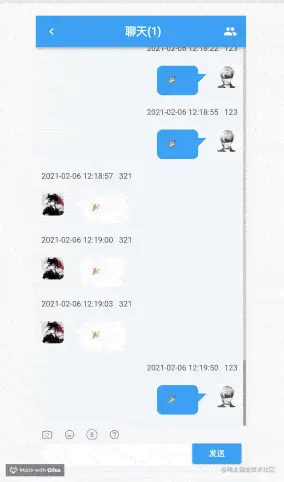### 结语

❤️关注+点赞+收藏+评论+转发❤️，原创不易，鼓励笔者创作更好的文章

• 关注后回复`简历`获取100+套的精美简历模板
• 关注后回复`好友`拉你进技术交流群+面试交流群，也可以和我讨论此次实践内容。
• 欢迎关注`秋风的笔记`